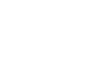How many hours, days, minutes, seconds in a month? - letsdiskussTrishna Dhanda

Self-Starter!!!!! | Posted | Education

# How many hours, days, minutes, seconds in a month?

1
0

Student | Posted

Ever thought how many seconds, minutes and hours are there in a month or how many months in a decade? Here is a small guide which will blow the minds with the exact numbers.

Seconds in a Month
Before getting deeper and seeing how many seconds are there in a month, it is necessary to understand the actual meaning of Second. It is a base unit of time but it is not considered as the smallest. The base units are those on which other units are based on. It means that all minutes, hours and even nanoseconds are based on the Second. As there are 365 days in a year and 366 in the leap year so according to 365 days there are approximately 2628003 seconds and as per leap year there are 31,622,400 seconds in a year.How many Minutes in a Month ?
A minute is defined as the unit of time which is usually equal to 1/60 of an hour or in simpler language, the 60 seconds. An approx value which is estimated for minutes in a month is 43800. When we talk about a leap year that is 366 days, therefore it is 527,050 minutes in a year.Hours in a Month
An hour is a period of time which is a unit of time. The number of minutes in an hour is 60 and there are 24 hours in a whole day. An estimated hours in a month is 730 hours
Hours are also an essential element to calculate the total work hours in a month of an employee or any person. There are many people who are paid on the basis of how many work hours they have. In simpler language, how many hours they have used their potential powers to be productive and have completed the work/task. There are fundamentally 2 ways by which work hours are calculated. As per the full-time employees, there are 130 hours in a month